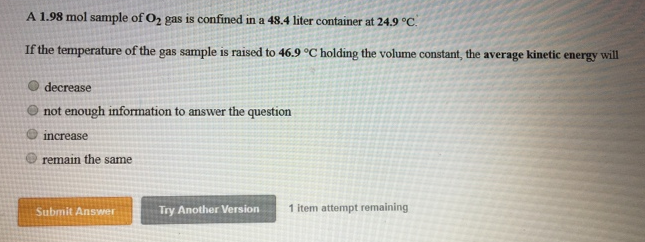# Problem: A 1.98 mol sample of O2 gas is confined in a 48.4 liter container at 24.9°C.If the temperature of the gas sample is raised to 46.9°C holding the volume constant the average kinetic energy will (i) decrease (ii) not enough information to answer the question (iii) increase (iv) remain the same

###### FREE Expert Solution
83% (472 ratings)###### Problem Details

A 1.98 mol sample of O2 gas is confined in a 48.4 liter container at 24.9°C.

If the temperature of the gas sample is raised to 46.9°C holding the volume constant the average kinetic energy will

(i) decrease

(ii) not enough information to answer the question

(iii) increase

(iv) remain the same